# Olympiad Test: Pattern - 2

## 20 Questions MCQ Test Mathematics Olympiad Class 7 | Olympiad Test: Pattern - 2

Description
Attempt Olympiad Test: Pattern - 2 | 20 questions in 40 minutes | Mock test for Class 7 preparation | Free important questions MCQ to study Mathematics Olympiad Class 7 for Class 7 Exam | Download free PDF with solutions
QUESTION: 1

### Direction: Choose the appropriate number which follow the given pattern.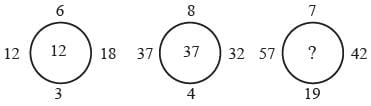Solution: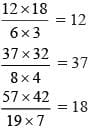QUESTION: 2

### Direction: Choose the appropriate number which follow the given pattern.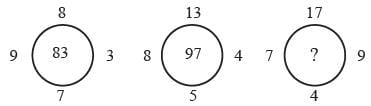Solution:

(8 × 7) + (9 × 3) = 56 + 27 = 83
(13 × 5) + (8 × 4) = 65 + 32 = 97
(17 × 4) + (7 × 9) = 68 + 63 = 131

QUESTION: 3

### Direction: Choose the appropriate number which follow the given pattern.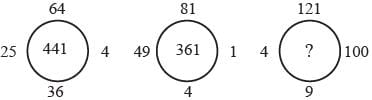Solution:

√64 + √25 + √36+ √4 = 8 + 5 + 6 + 2
= 21 → 212 = 441
81 + 49 + 4+ 1 = 9 + 7 + 2 + 1
= 19 → 192 = 361
√121 + √4 + √9+ √100 = 11 + 2 + 3 + 10
= 26 → 262 = 676

QUESTION: 4

Direction: Choose the appropriate number which follow the given pattern.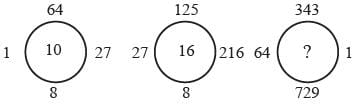Solution:

3√64 + 3√1 + 3√8 +3√27 = 4 + 1 + 2 + 3 = 10
3√27 + 3√8 +3√216 + 3√125 = 3 + 2 + 6 + 5
= 16
3√64 +3√729 + 3√1 + 3√343 = 4 + 9 + 1 + 7 = 21

QUESTION: 5

Direction: Choose the appropriate number which follow the given pattern.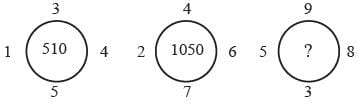Solution:

(12 + 52 + 42 + 32) × 10 = 510
(22 + 72 + 62 + 42) × 10 = 1050
(52 + 32 + 82 + 92)  × 10 = 1790

QUESTION: 6

Direction: Choose the appropriate number which follow the given pattern.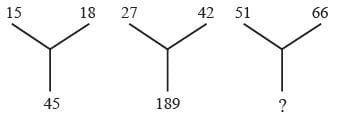Solution: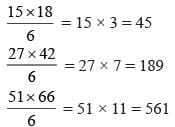QUESTION: 7

Direction: Choose the appropriate number which follow the given pattern.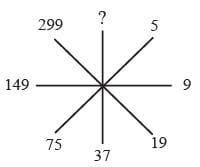Solution:

5 × 2 – 1 = 9;
9 × 2 + 1 = 19
19 × 2 – 1 = 37
37 × 2 + 1 = 75
75 × 2 – 1 = 149
149 × 2 + 1 = 299
299 × 2 – 1 = 597

QUESTION: 8

Direction: Choose the appropriate number which follow the given pattern.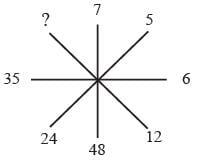Solution:

72 – 1 = 48
52 – 1 = 24
62 – 1 = 35
122 – 1 = 143

QUESTION: 9

Direction: Choose the appropriate number which follow the given pattern.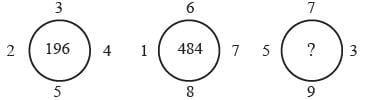Solution:

3 + 2 + 5 + 4 = 14 → 142 = 196
6 + 1 + 8 + 7 = 22 → 222 = 484
5 + 9 + 3 + 7 = 24 → 242= 576

QUESTION: 10

Direction: Choose the appropriate number which follow the given pattern.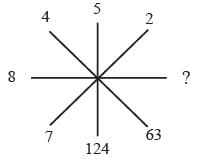Solution:

43 – 1 = 63
23 – 1 = 7
53 – 1 = 124
83 – 1 = 511

QUESTION: 11

Direction: Choose the appropriate number which follow the given pattern.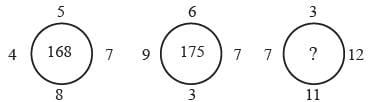Solution:

(4 + 8 + 7 + 5) × 7 = 24 × 7 = 168
(9 + 3 + 7 + 6) × 7 = 25 × 7 = 175
(7 + 11 + 12 + 3) = 33 × 7 = 231

QUESTION: 12

Direction: Choose the appropriate number which follow the given pattern.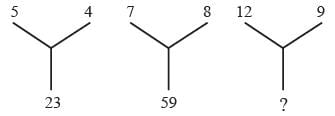Solution:

(5 ×  4) + 3 = 23
(7 × 8) + 3 = 59
(12 × 9) + 3 = 111

QUESTION: 13

Direction: Choose the appropriate number which follow the given pattern.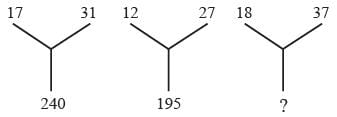Solution:

(17 + 31) × 5 = 240
(12 + 27) × 5 = 195
(18 + 37) × 5 = 275

QUESTION: 14

Direction: Choose the appropriate number which follow the given pattern.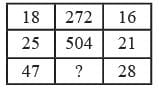Solution:

(18 – 1) × 16 = 272
(25 – 1) × 21 = 504
(47 – 1) × 28 = 1288

QUESTION: 15

Direction: Choose the appropriate number which follow the given pattern.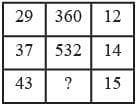Solution:

(29 + 1) × 12 = 360
(37 + 1) × 14 = 532
(43 + 1) × 15 = 660

QUESTION: 16

Direction: Choose the appropriate number which follow the given pattern.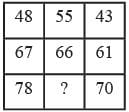Solution:

(48 – 43) × 11 = 55
(67 – 61) × 11 = 66
(78 – 70) × 11 = 88

QUESTION: 17

Direction: Choose the appropriate number which follow the given pattern.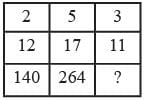Solution:

(5 – 2) × 1 + 0 = 3
(17 – 12) × 2 + 1 = 11
(264 – 140) × 3 + 2 = 374

QUESTION: 18

Direction: Choose the appropriate number which follow the given pattern.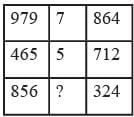Solution:

(9 + 7 + 9) – (8 + 6 + 4) = 25 – 18 = 7
(4 + 6 + 5) – (7 + 1 + 2) = 15 – 10 = 5
(8 + 5 + 6) – (3 + 2 + 4) = 19 – 9 = 10

QUESTION: 19

Direction: Choose the appropriate number which follow the given pattern.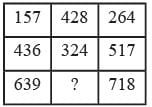Solution:

(264 – 157) × 4 = 428
(517 – 436) × 4 = 324
(718 – 639) × 4 = 316

QUESTION: 20

Direction: Choose the appropriate number which follow the given pattern.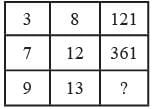Solution:

3 + 8 = 11 → 112 = 121
7 + 12 = 19 → 192 = 361
9 + 13 = 22 → 222 = 484Use Code STAYHOME200 and get INR 200 additional OFF Use Coupon Code